Courses

# Test: Number Series Type 1 & Type 2

## 10 Questions MCQ Test Logical Reasoning (LR) and Data Interpretation (DI) | Test: Number Series Type 1 & Type 2

Description
This mock test of Test: Number Series Type 1 & Type 2 for CAT helps you for every CAT entrance exam. This contains 10 Multiple Choice Questions for CAT Test: Number Series Type 1 & Type 2 (mcq) to study with solutions a complete question bank. The solved questions answers in this Test: Number Series Type 1 & Type 2 quiz give you a good mix of easy questions and tough questions. CAT students definitely take this Test: Number Series Type 1 & Type 2 exercise for a better result in the exam. You can find other Test: Number Series Type 1 & Type 2 extra questions, long questions & short questions for CAT on EduRev as well by searching above.
QUESTION: 1

### Directions(Q1-10): Find out the missing number in the following series. 27, 32, 30, 35, 33, __.

Solution: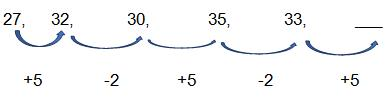33 + 5 = 38

QUESTION: 2

### 12000, 2395, 474, 89.8, ___.

Solution:

12000/5 - 5 = 2400 - 5 =2395
2395/5 - 5 =479 – 5 = 474
474/5   - 5 = 94.8 - 5 = 89.8
89.8/5 – 5 = 17.96 – 5 = 12.96

QUESTION: 3

### 1, 2, 8, 33, 148, __.

Solution:

1 * 1 + 1^2 = (1 + 1) = 2
2 * 2 + 2^2 = (4 + 4) = 8
8 * 3 + 3^2 = (24 + 9) = 33
33 * 4 + 4^2 = (132 + 16) = 148
148 * 5 + 5^2 = (740 + 25) = 765.

QUESTION: 4

34, 18, 10, ___

Solution: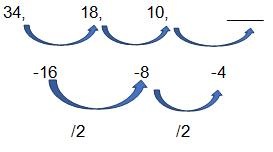QUESTION: 5

17, __, 65, 113, 129, 161, 209.

Solution:

The sequence is +16, +32, +48, +16, +32,……
So, term is 33.

QUESTION: 6

100, 70, 46, 28, __, 10

Solution:

The sequence is –(6*5), -(6*4), -(6*3), -(6*2),
So, term is 16.

QUESTION: 7

8, 24, 12, ___, 18, 54

Solution: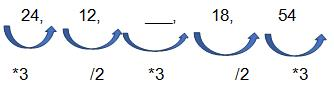So, term is 12*3 = 36.

QUESTION: 8

0, 4, 18, 48, ___, 180

Solution: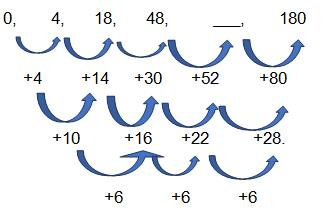So, term is 100.

QUESTION: 9

7, 9, 13, 21, 37, ____

Solution: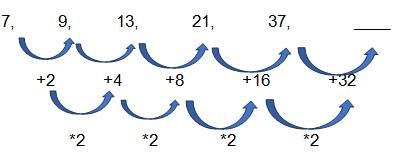So, term is 69

QUESTION: 10

1, 8, 28, 99, 412, 2075, ____.

Solution:

1*1 + 7*1 = 1 + 7 = 8
8*2 + 6*2 = 16 + 12 = 28
28*3 + 5*3 = 84 + 15 = 99
99 * 4 + 4 * 4 = 396+ 16 = 412
412 * 5 + 3 * 5 = 2060 + 15 = 2075
2075 * 6 + 2 * 6 = 12450+12 = 12462